# SAT Math Multiple Choice Question 356: Answer and Explanation

### Test Information

Question: 356

11.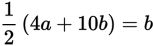If (a, b) is a solution to the equation above, what is the ratio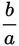, given that a ≠ 0?

• A. -3
• B. -2
• C.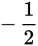• D.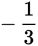Strategic Advice: When you're given only one equation but two variables, chances are that you can't actually solve the equation (unless one variable happens to cancel out), but rather that you are going to need to manipulate it to look like the desired expression (which in this question is).
Getting to the Answer: This type of question can't be planned out step-by-step-instead, start with basic algebraic manipulations and see where they take you. First, distribute the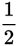on the left side of the equation to get 2a + 5b = b. There are two terms that have a b, so subtract 5b from both sides to get 2a = -4b. You're hoping for plain b in the numerator, so divide both sides by -4 to get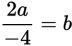. Finally, divide both sides by a to move the a into a denominator position under b. The result is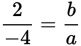, which means the ratiois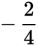, or.